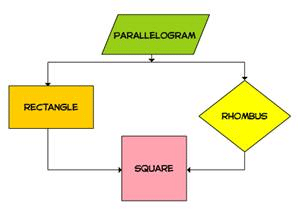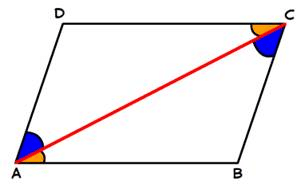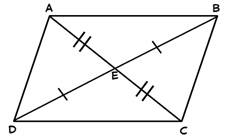Parallelogram - Class 8

# Parallelogram - Class 8 Notes - Class 8

## Document Description: Parallelogram - Class 8 for Class 8 2022 is part of Class 8 preparation. The notes and questions for Parallelogram - Class 8 have been prepared according to the Class 8 exam syllabus. Information about Parallelogram - Class 8 covers topics like and Parallelogram - Class 8 Example, for Class 8 2022 Exam. Find important definitions, questions, notes, meanings, examples, exercises and tests below for Parallelogram - Class 8.

Introduction of Parallelogram - Class 8 in English is available as part of our Class 8 preparation & Parallelogram - Class 8 in Hindi for Class 8 courses. Download more important topics, notes, lectures and mock test series for Class 8 Exam by signing up for free. Class 8: Parallelogram - Class 8 Notes - Class 8

Needed a Document for parellogram?
Ref: https://edurev.in/question/508827/Needed-a-Document-for-parellogram-

 1 Crore+ students have signed up on EduRev. Have you?

A quadrilateral is a closed figure which has four sides, four angles and four vertices. There are different types of quadrilaterals such as Parallelogram, Rectangle, Square, Rhombus, Trapezium and Kite.

A parallelogram is a quadrilateral in which
•  opposite sides are parallel and equal,
•  opposite angles are equal,
•  the diagonals bisect each other,
•  each diagonal divides it into two congruent triangles,
•  adjacent angles are supplementary.

A square is a parallelogram in which
•  all the sides are equal,
•  each angle measures 90°,
•  diagonals are equal and bisect at right angles.

A rectangle is a parallelogram in which
•  diagonals are equal and bisect each other,
•  each angle measures 90°.

A rhombus is a parallelogram in which
•  all four sides are equal,
•  diagonals bisect each other at right angles.A diagonal of a parallelogram divides it into two congruent triangles.If ABCD is a parallelogram and AC is a diagonal, then ΔABC ≅ ΔCDA.

If each pair of opposite sides is equal in a quadrilateral, then it is a parallelogram.In a Quadrilateral ABCD, If AB = CD and BC = AD then, it is a parallelogram.

If the diagonals of a quadrilateral bisect each other, then it is a parallelogram.In a Quadrilateral ABCD in which diagonals bisect each other then ABCD is a parallelogram.

The document Parallelogram - Class 8 Notes - Class 8 is a part of Class 8 category.
All you need of Class 8 at this link: Class 8
 Use Code STAYHOME200 and get INR 200 additional OFF

Track your progress, build streaks, highlight & save important lessons and more!

,

,

,

,

,

,

,

,

,

,

,

,

,

,

,

,

,

,

,

,

,

;